Kyle Heldman NSF REU Summer 2009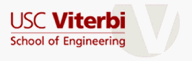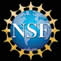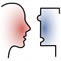Post-Stroke Assistive Robotics Pioneer and Bandit Robot Configuration Movement Analysis

 Motivation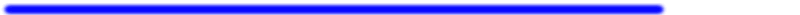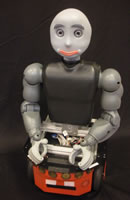This project lies in the post-stroke rehabilitation domain and focuses on social human-robot interaction in order to better provide the necessary therapy to patients. While the upper torso Bandit robot tries to administer post-stroke therapy to patients, a necessary interaction and life-like movement is required. This type of movement requires smooth transitions and travel of the robot in order to emulate human behavior and obtain positive reactions and results from the patients themselves.

 MethodsWhen developing the method to solve the robot movement problem, a more general approach was taken in order to obtain both individual robot configuration results as well as a more applicable process to apply to other robot configuration and movement requirements. In order to achieve this greater applicability, a motor model and testing was done. In addition a center of mass model was made in order to find the system center of mass for any unique robot configuration. Combining these different models, a more general analysis of any Pioneer base and upper torso Bandit robot configuration was achieved. An example of the velocity profiles used to test the Pioneer base robot can be seen in Figure 1. An example center of mass model for a 56" upper torso Bandit robot can be seen in Figure 2.

 Figure 1 - Test Velocity Profiles Figure 2 - Center of Mass Model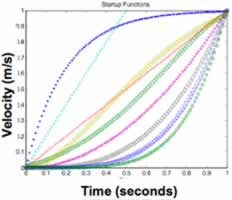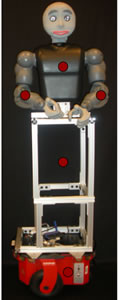ImplementationThe motors that turn the wheels on the Pioneer base robot base are two Pittman 19.7:1 ratio DC motors. After an initial motor model was found, a series of nine different velocity profiles, which can again be seen in Figure 1 above, were used to test the motors. Using these velocity profiles, an appropriate velocity profile was found that allowed for smooth Pioneer base and upper torso Bandit robot movement while staying within the limitations of the other robotic hardware and software components.
For each possible component in the Pioneer and Bandit system, a series of tipping and weight tests were done in order to calculate each component’s center of mass. This center of mass model was calculated to be a single mass point in a three dimensional plane. By finding the center of mass of each individual component, the overall center of mass could then be calculated regardless of arrangement of system. This center of mass implementation allows for robot design change and better accuracy. This lead to the calculation of maximum acceleration and deceleration of the particular configuration in which the robot remains stable and does not tip.
Figure 3 below shows the final results of the velocity profile and center of mass tests. The velocity profile y = x^4 was used on the robot configuration until maximum acceleration or deceleration was met, in which case the robot follows the y = MaxAcc•x curve. Figure 4 below shows actual results of this type of velocity curve movement. The results show that the robot very accurately follows the given velocity commands and demonstrated smooth movement and transitions.

 Figure 3 - Theoretical Results Figure 4 - Actual Results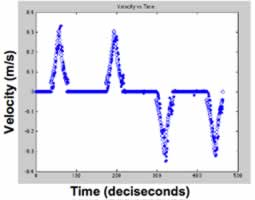ApplicationUsing the motor and center of mass model, an accurate velocity profile and maximum acceleration was found for the configuration in Figure 2. The applicability of these two models is widespread since they can be used in any configuration. Figure 2 represents the approximate points found in the system for the center of mass model. Figure 3 shows the theoretical velocity profile for which any robot configuration would take. The robot would travel along the function y = x^4 until meeting the y=MaxAcc•X function. At this point, the robot travels along this linear function to avoid acceleration over its maximum limit. In demonstration, the different robot configurations performed best with the y = x^4 velocity profile on both startup and slow down. The results and diagrams from the various tests prove that the center of mass and motor models are accurate. Because of their widespread applicability, these models and procedures can be further implemented and used on any robot configuration in the lab in future experiments whenever smooth motion is required.

 Work Done Under: Dr. Maja Mataric' Dr. Eric WadeContact: Kyle Heldman